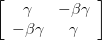# Of Rotation, LT and Acceleration

This post uses Easy LaTeX Pro to display equations.

Lorentz transformation is a rotation in the Minkowski space. In order to see it, let’s first look at rotation in Euclidean space, which can be written as X= R X. In the 2-D case, the matrix of rotation R is,$\left[ {\begin{array}{*{20}c}{\cos \theta } & { - \sin \theta } \\{\sin \theta } & {\cos \theta } \\\end{array}} \right]$
So, the matrix equation expands towhere$\theta$ is the angle of rotation. This is how a point$X=(x,y)$ in the original frame transforms toin the rotated frame.

Similarly, LT in Minkowski space is X= L X. Lorentz Transformation matrix (in our 2-D case) is,$\left[ {\begin{array}{*{20}c}\gamma & { - \beta \gamma } \\{ - \beta \gamma } & \gamma \\\end{array}} \right]$
where$\beta = v/c$ and$\gamma = 1/\sqrt {1 - \beta ^2 }$

This expands toNote that rotation (and so LT) is a linear transformation, which means that the matrix R (or L) has to be independent of the vector it transforms. What happens when the matrix is a function of x, y or t? The geometry becomes non-flat and the metric tensor we defined doesn’t define the invariant distance any longer. The geometry requires a different metric tensor. Therefore, rotation or LT as we defined it and the associated single component equations is not valid any more. I will illustrate it further using 2-D rotation in the next post and show what they mean when they say that space-time is curved.

Continued